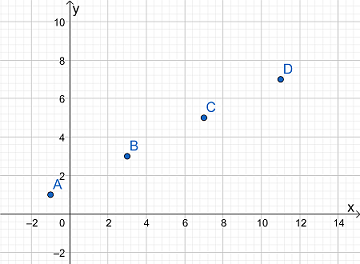# Set up a T-table, using t= 0, 1, 2, and 3. Plot the points. Write one equation by eliminating the...

## Question:

Set up a T-table, using {eq}t= 0, 1, 2, {/eq} and {eq}3. {/eq} Plot the points. Write one equation by eliminating the parameter. Describe the resulting curve. {eq}x = 4t - 1 \,\, y = 2t + 1 {/eq}

## Parametric Equation:

A parametric curve in the x-y plane is defined by the set of equations:

{eq}x=x(t) \\ y=y(t). {/eq}

The graph of the parametric equation is obtained by plotting the points {eq}(x,y) {/eq}

when the parameter t varies.

We are given the parametric equation

{eq}x = 4t - 1 \\ y = 2t + 1. {/eq}

The values of x and y for {eq}t= 0, 1, 2, 3 {/eq} are reported in the table below.

{eq}t=0 \Rightarrow x = - 1, \; y= 1 \\ t=1 \Rightarrow x = 3, \; y= 3 \\ t=2 \Rightarrow x = 7, \; y= 5 \\ t=3 \Rightarrow x = 11, \; y= 7. {/eq}

The points are plotted in the figure below.

The cartesian equation of the curve is found by eliminating the parameter t.

We evaluate the parameter t from first equation and plug it into the second equation

{eq}\displaystyle t = \frac{1}{4}x + \frac{1}{4} \\ \displaystyle y = 2(\frac{1}{4}x + \frac{1}{4}) + 1 = \frac{1}{2}x + \frac{5}{4}. {/eq}